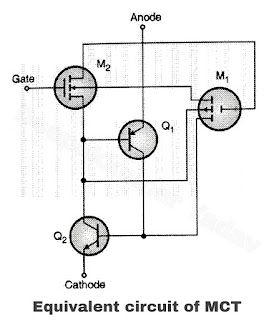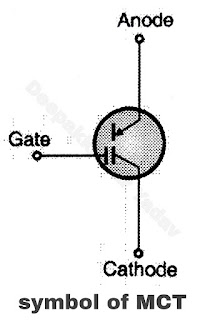# Working of MOS Controlled Thyristor

MOS Controlled Thyristor (MCT)

Construction of MOS Controlled Thyristor (MCT)

• MCT is a special device which has the characteristics of a four layer p-n-p-n SCR and a MOS - gate structure. The schematic diagram of an MCT is shown in Figure (a). Its equivalent circuit is shown in Figure (b) and circuit symbol in Figure (c).Figure (a) - schematic diagram of an MCTFigure (b) - Equivalent circuit of MCTFigure (c) - Symbol of MCT
• The pnpn structure of MCT has been represented by two transistors, one pnp (Q1) and other npn (Q2) transistors and the MOS - gate structure can be bong represented by a p-channel MOSFET Mand n-channel MOSFET M2.
• Note that the structure of MCT is n-p-n-p and not p-n-p-n like SCR. Therefore the anode will act as reference point and not the cathode. The gate signals are applied with respect to anode.
• MCT turn on : If initially MCT is in the off state and a negative gate voltage VGA is applied, then the p-channel MOSFET M1 in the equivalent circuit will be turned on. It then provides the base current for the npn transistor Q2. Due to the regenerative action that takes place between Q1 and Q2 (just like that in SCRs), the MCT turns on and gets latched.
• MCT turn off : If the MCT is in conduction and a positive gate voltage VGA is applied, then the n-channel MOSFET M2 in the equivalent circuit is turned on. The drain current of M2 diverts away the base current of Q1. So all the collector current of Q2 does not act as base current of Q1. Hence regeneration does not continue and MCT turns off.